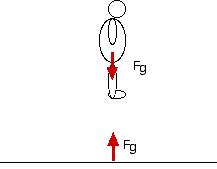Action-Reaction

May the ground rise to meet you.

Introduction

When you jump off a chair, you fall towards the earth, but the earth also rises to meet you.The earth exerts a force on the falling person, the person exerts an equal and opposite force on the earth.

To Do and Notice

Carefully stand on a chair about 1 meter above the ground.

Jump off and fall to the ground. Be sure to absorb the shock of hitting the ground by bending your knees.

Notice that as you fell toward the earth, the earth rose to meet you.

What's Going On?

The two forces are an action-reaction pair.

The forces are on different objects, one force is on the person and the the other is on the earth.

This is important, action reaction pairs are always on different objects.

Math Root

For a 60 kg person the force of gravity from the earth is Fg = mg = 60 * 10 = 600 N

where g is the acceleration of gravity, approximately 10 m/s^2

The force of the earth on the person and of the person on the earth is an action-reaction pair, so these forces are equal and opposite.

The acceleration of the person is a = F/m = 600 N/60 kg = 10 m/s^2

The acceleration of the earth is a = F/M = 600 N/ 6 X 10^24 kg = 10^-22 m/s^2

The distance the person falls is about 1 m.

The earth rises for the same time that the person falls, and

d = 1/2 a t^2

so since the time of fall is the same for the person and the earth, the distance moved is proportional to the acceleration.

dearth/dperson = aearth/aperson

The distance the earth moves is d earth = 1m * 10^-22/10 = 10^-23 m

This is 100 million times smaller than the diameter of a nucleus.

 Scientific Explorations with Paul Doherty © 2007 30 January 2007